# How to Find Duplicate Values in Excel

You can Find duplicate values in Excel by reading this blog. An Excel sheet is a great way of packing lots of data into a dense area. But, these documents will become unmanageable and unwieldy when they’re in use for a long period of time. This example teaches you how to find duplicates in Excel. You can also learn this from our Excel Courses online for free.

In this tutorial, we will figure out How to Find Duplicate values in an Excel sheet using two ways. To begin with, we will figure out how to use Conditional Formatting to highlight the copy cells. Then, we will show how to use the Excel Countif Function to find the duplicates. Now, we are not using a formula to find duplicates in excel. But how conditional formatting and countif function helps.

## How to Find Duplicate value using Conditional Formatting!

If you want to find duplicate values in excel then kindly follow below step by step procedure and easily get duplicate values in Excel or Spreadsheets.

1. Select the range of cells you wish to test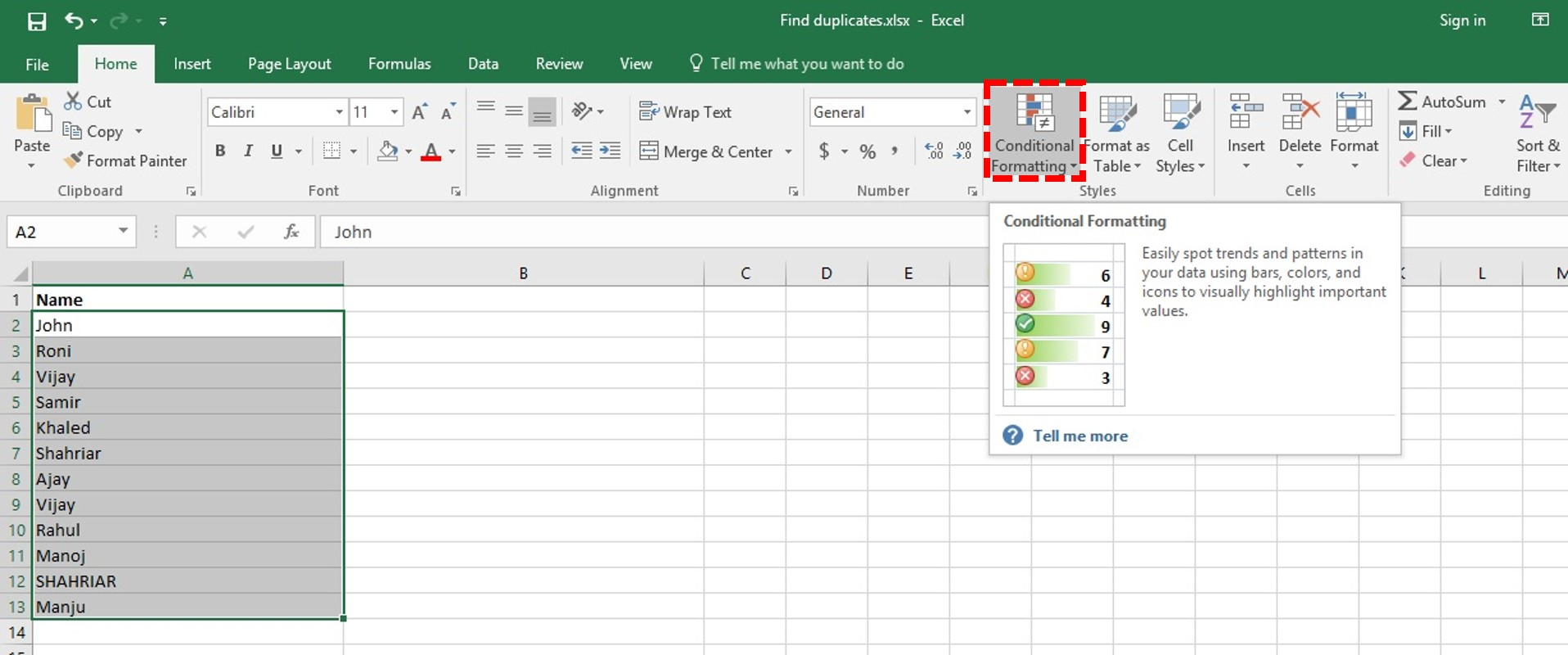2. Click on Home Tab
3. Click on Conditional Formatting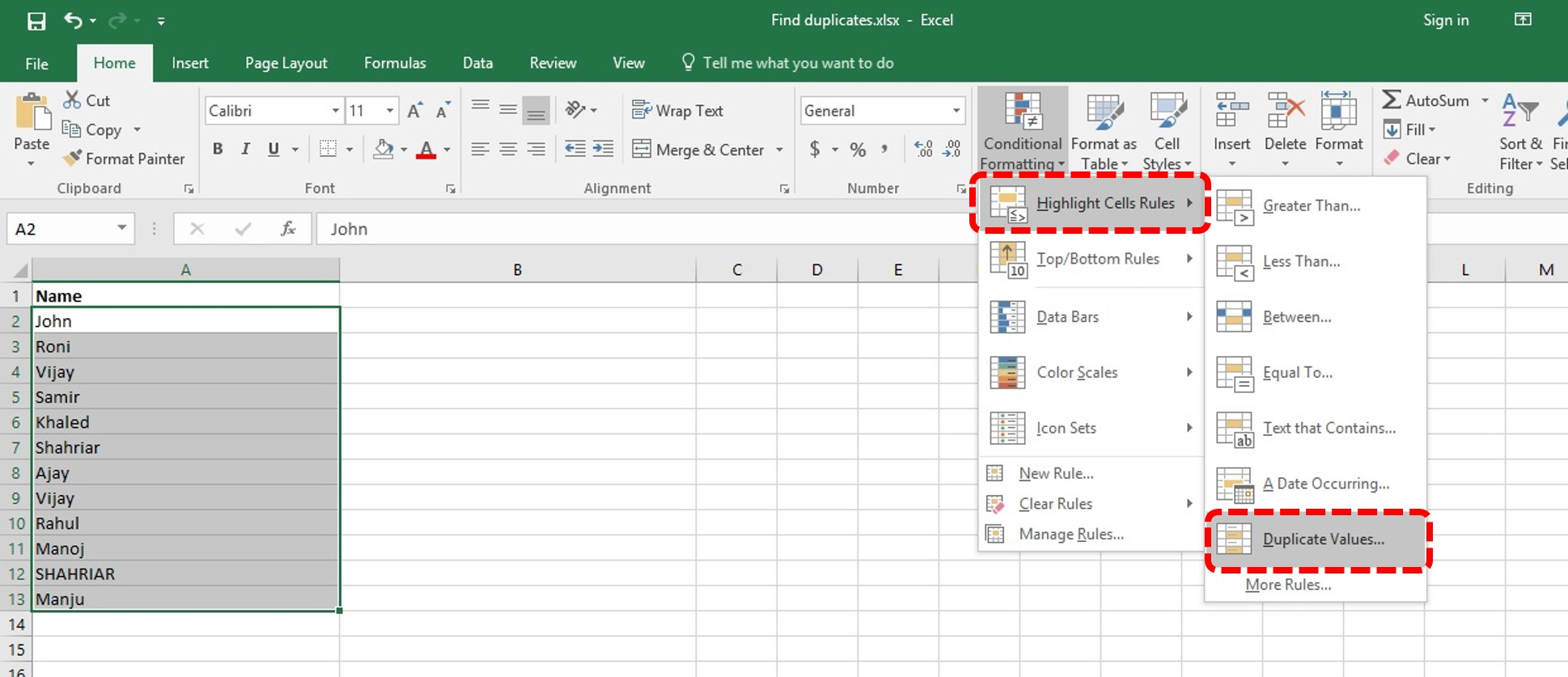4. Select the Highlight Cells Rules option
5. Then select the Duplicate Values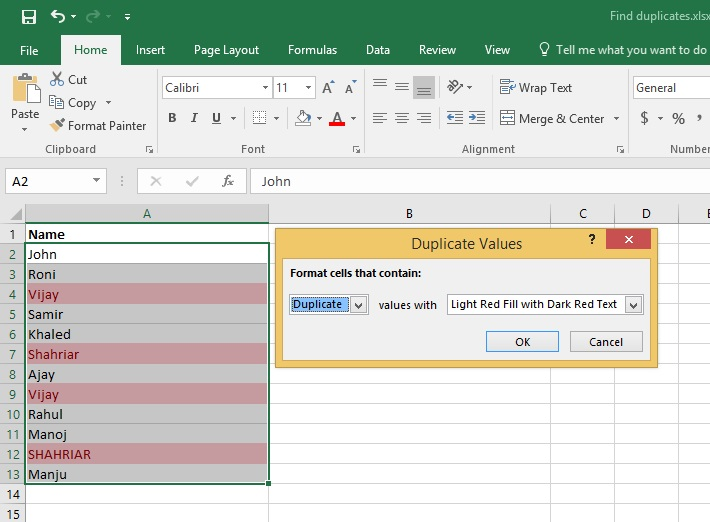6. Selected Column will Highlight Duplicate Values.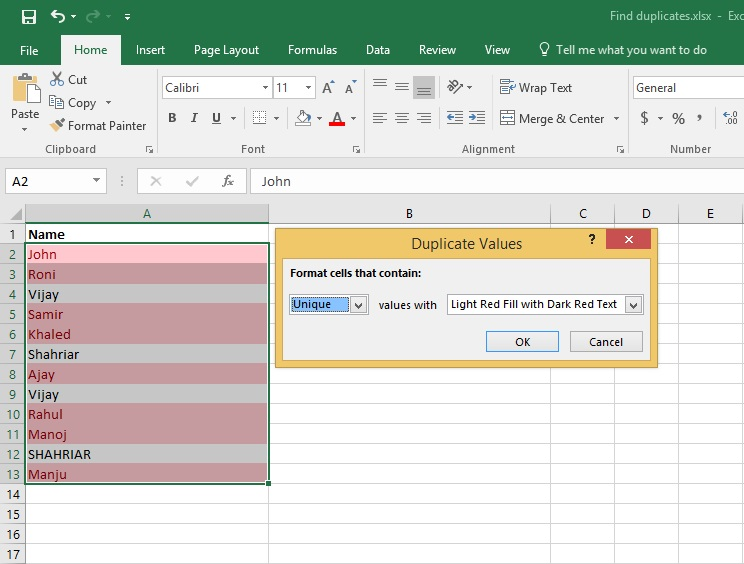7. Duplicate Column list will now get identified

Note: If we select Unique from the list. So, the previous selection is reversed. We are finding Duplicate values and not Unique values. So, select Duplicate from the drop-down list.

Then Click OK.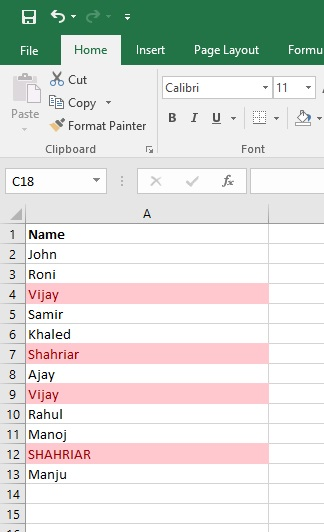So, we have learned how to find duplicate values using Conditional Formatting. You can learn such Excel Tips & Tricks in Yoda Learning

Note: This method will only work if cell contents are less than 256 characters in length. This is because Excel functions cannot handle text strings that are longer than this

## Find duplicate using COUNTIF Function

Using the COUNTIF function helps us:

1. Find only the duplicate values, and
2. Count how many times the value is repeated in the list.

### So let’s find out a duplicate with Countif Function in Excel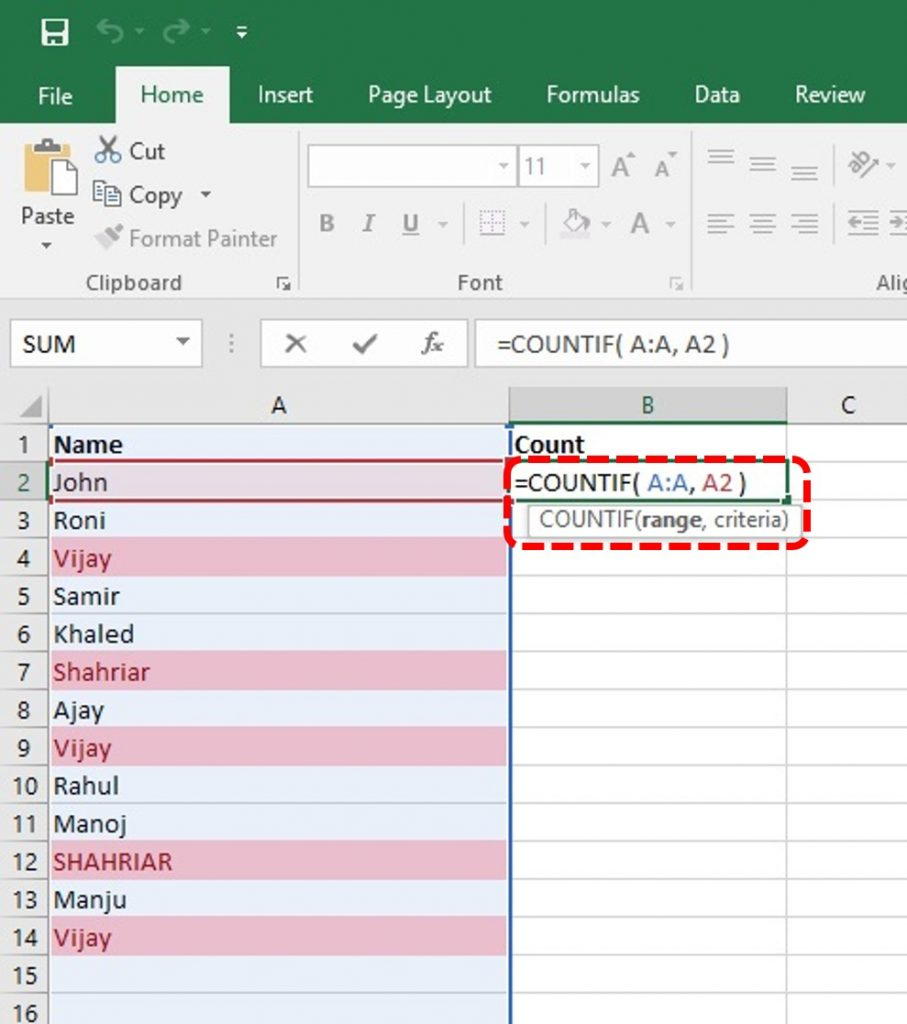Now let see how CountIF calculates the number of repetition.

1: Now in Column B we will count each name repetition. In cell B1 type the name of Column Count as like above picture. Now use below formula in cell B2 for count the number of repetition of the name John in cell A2:

=COUNTIF( A:A, A2 )

2: This formula is telling to count the repeated number of the value of cell A2 in the range of A:A (means the whole column of A).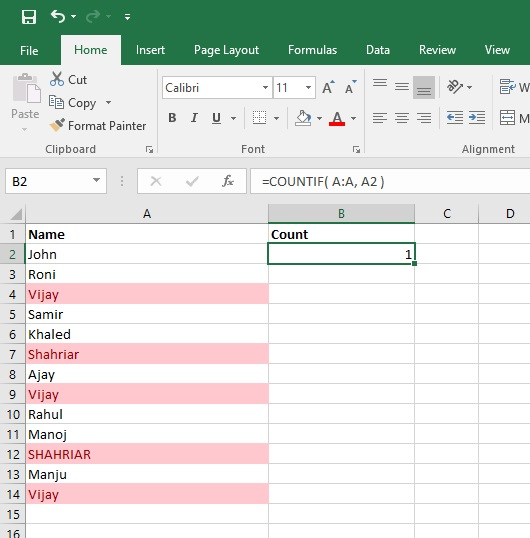Now we can see in cell B2, it counts that name John is entered only 1 time. So now let input the same formula into other cells of column B.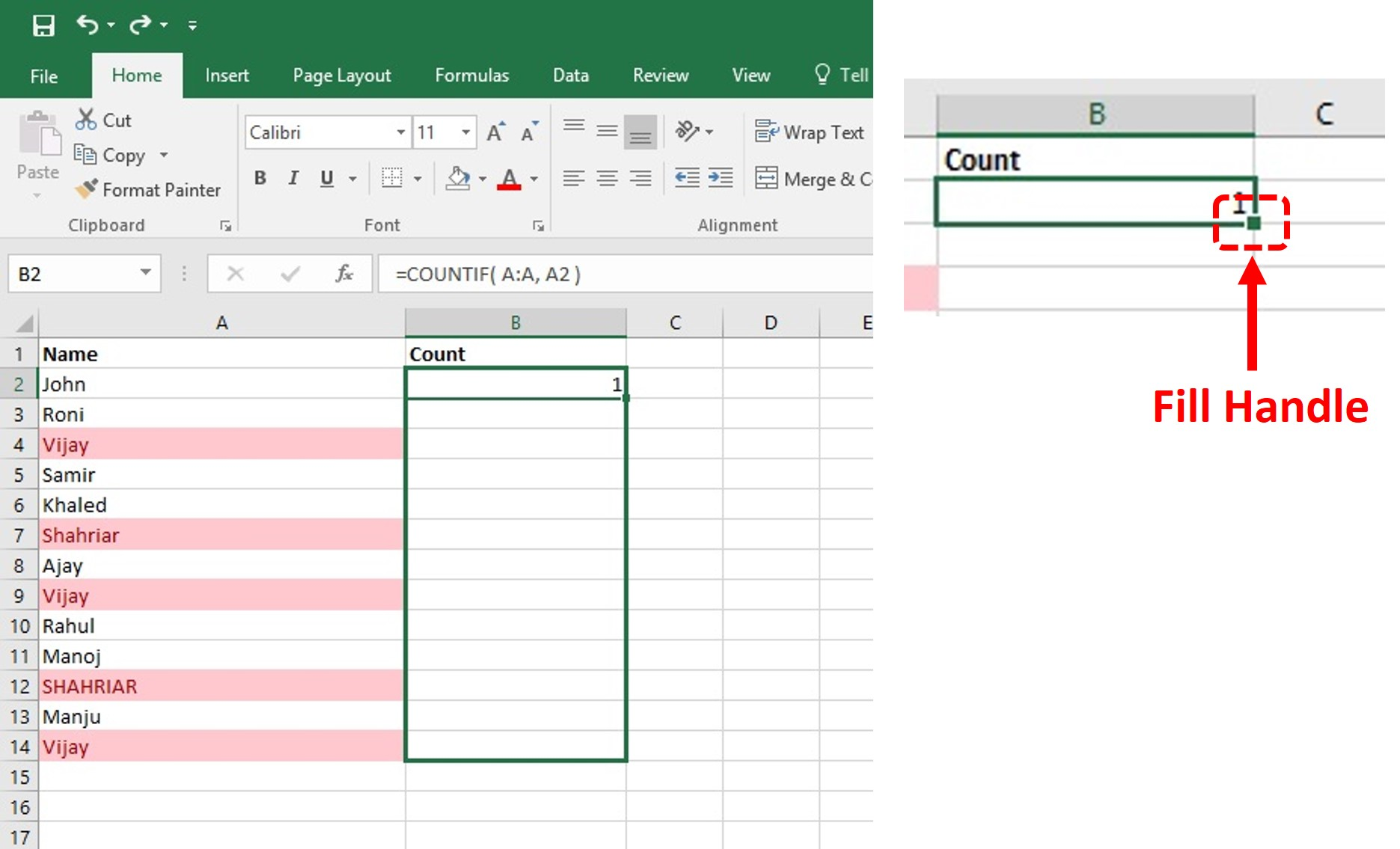3: Using the Fill handle of the selected cell. Click, hold, and drag it below another cell of column B as like the above picture. The formula will be copied automatically for other cells of column B.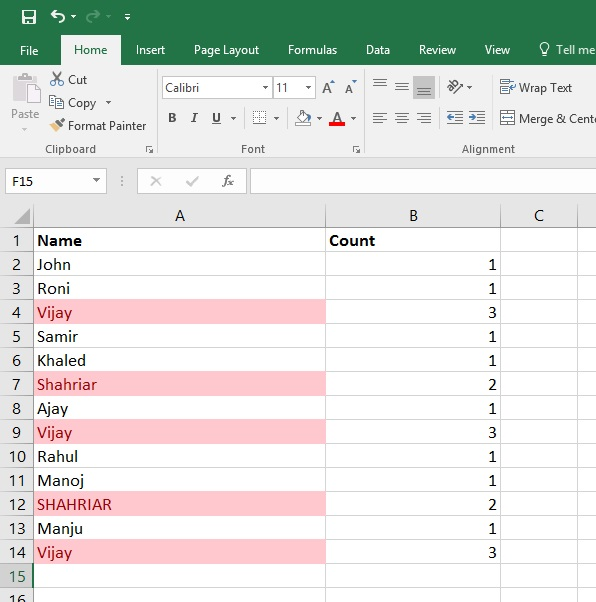4: Now column B is showing the number of entries of each name. We typed Vijay 3 times and it is also calculated by our COUNTIF function.

So now we have successfully learned those two ways to find duplicate values in the excel sheet.

Check out our highly recognized Excel Ninja Training Program and become a Hero at the Office. The comprehensive training provides the best in premium quality videos with a recognized certification.

## 1 Comment

•